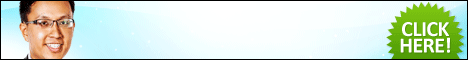## How To Calculate Compound Interest If You Don’t Have A Financial Calculator…

Tue, Nov 15, 2011

Investment

Example, you are an investor. You  invest your \$10,000 in a financial instrument, giving you 9% interest, compounded on annual basis for 10 years. How much will you get, at the end of the 10 years?

If you are a financial planner, like me, you will definitely reach out your financial calculator and punch in these figures, to get the answers. idoiteveryday

Set: End
n: 10
i%: 9
PV: -10,000
FV: Solve

…..and in an instance, you will get \$23,673.63

…………………………………………………………………………………………………………………………………………………………………………………….
…………………………………………………………………………………………………………………………………………………………………………………….

In my “financial planning for wealth manager” (catered for high networth individual) class, I learnt to calculate the answers, without using a financial calculator. You can use a normal, scientific calculator to get the answers.

How do you do that?

You can calculate it this way…..Use this formula….. Principal (1+interest)number of years

Just take 10,000 (1+0.09)10  = \$23,673.63

Easy right?

For now, just remember this formula. In the next blog post, I will share with you the intricate mechanics of this formula. Share this blog post, if you find it useful! 🙂